# 混合模式

• 可简单理解为 指上下层图片相互有重叠时如何取色的一种称呼

• 以下是百科给的解释 但我们今天要说的是Unity中的颜色混合

# Blend

• 常用的混合模式通过Blend实现 如下所示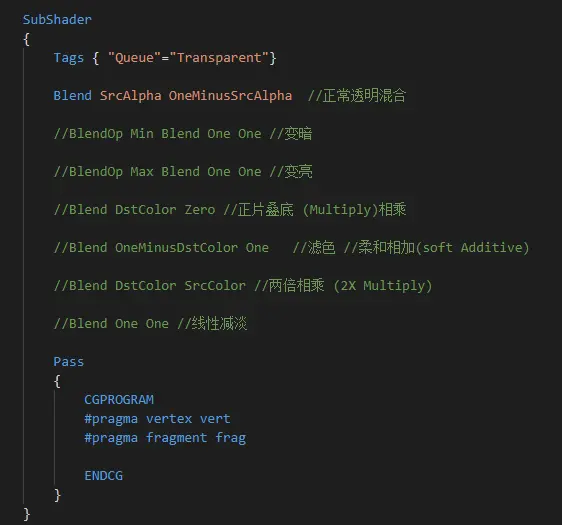• 以下为测试图片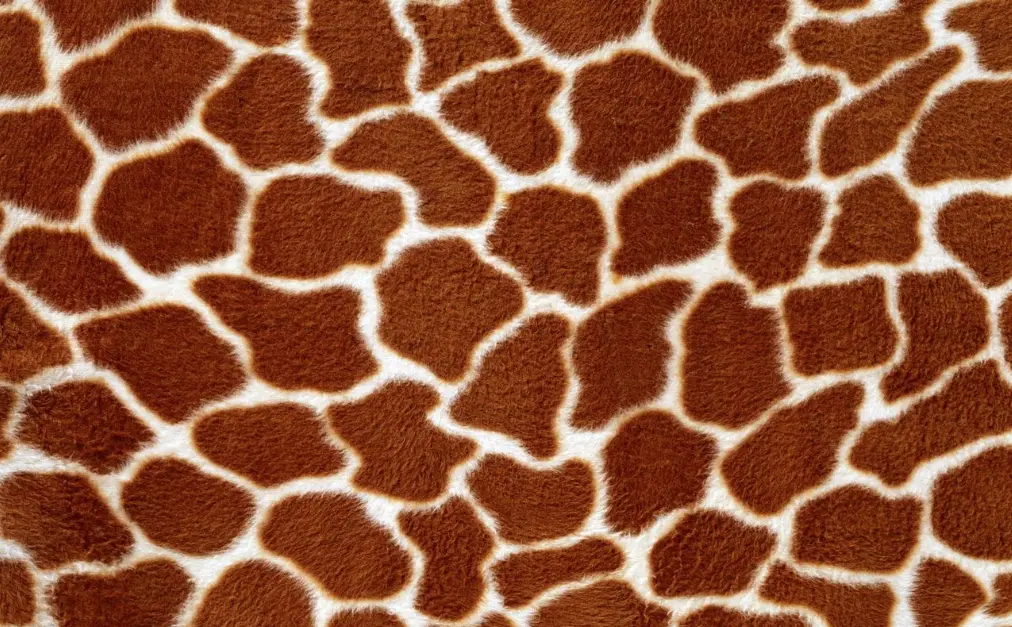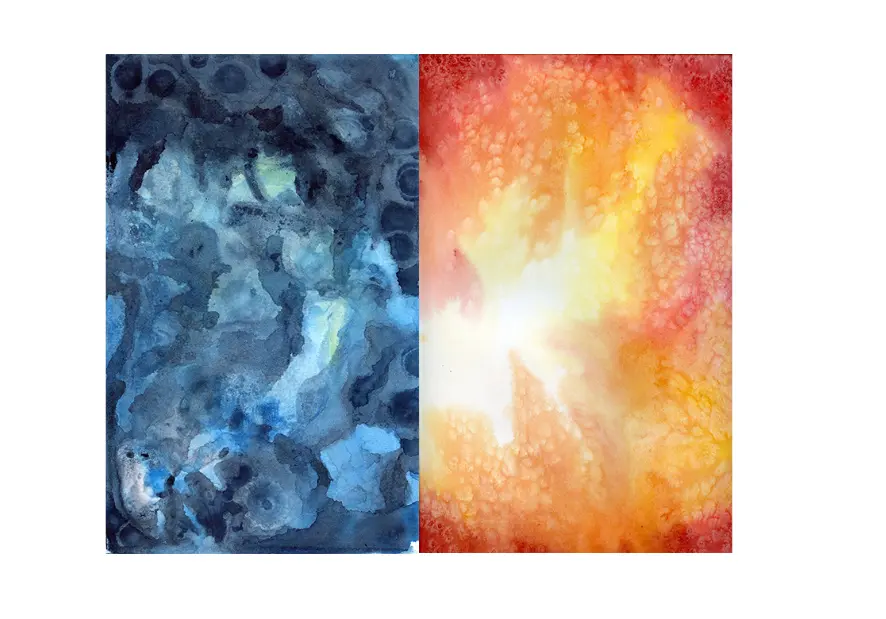• 常用的滤色混合效果如下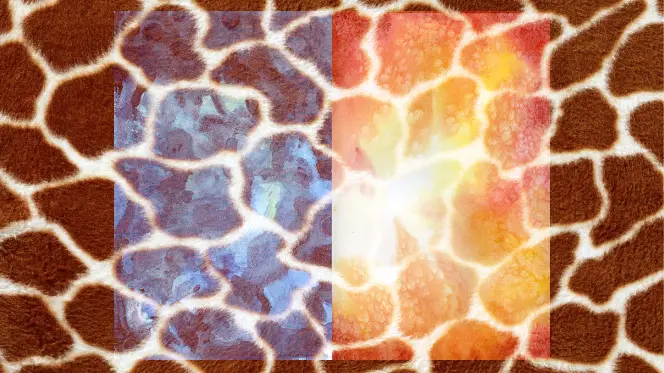# 代码实现混合模式

• 可以看到Blend已经可以实现很多种混合效果 但这也只是混合模式的一部分

• 框架代码如下 还是前几篇文章一样 只更改frag中代码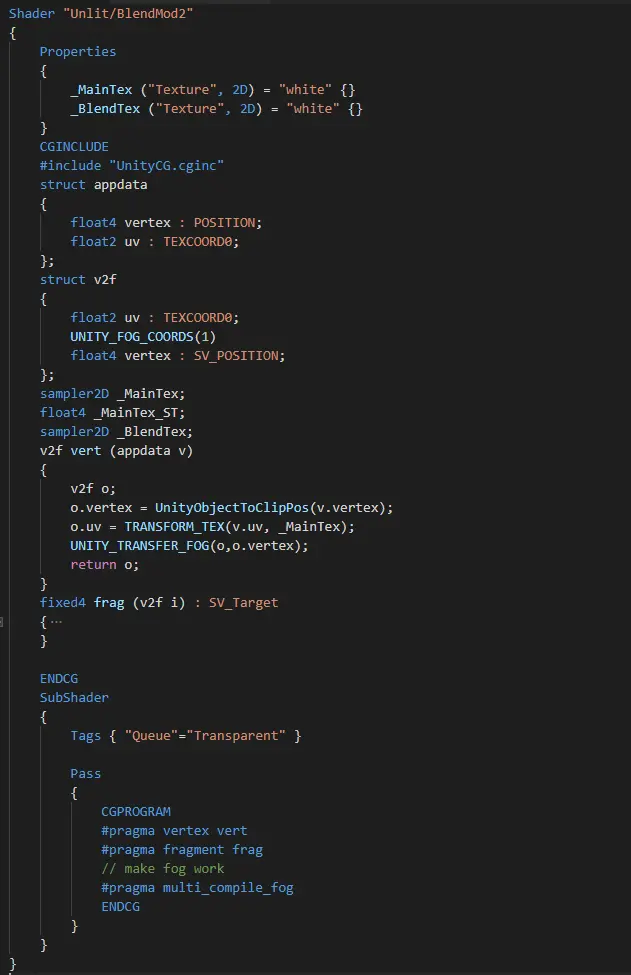• 其中MainTex为底图 BlendTex为要混合的图片

• 颜色取值为（0-1） A为底图颜色 B为混合图颜色 C为输出图颜色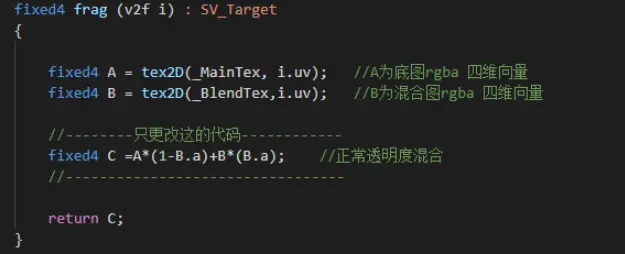frag函数代码
• 使用step()函数来代替if判断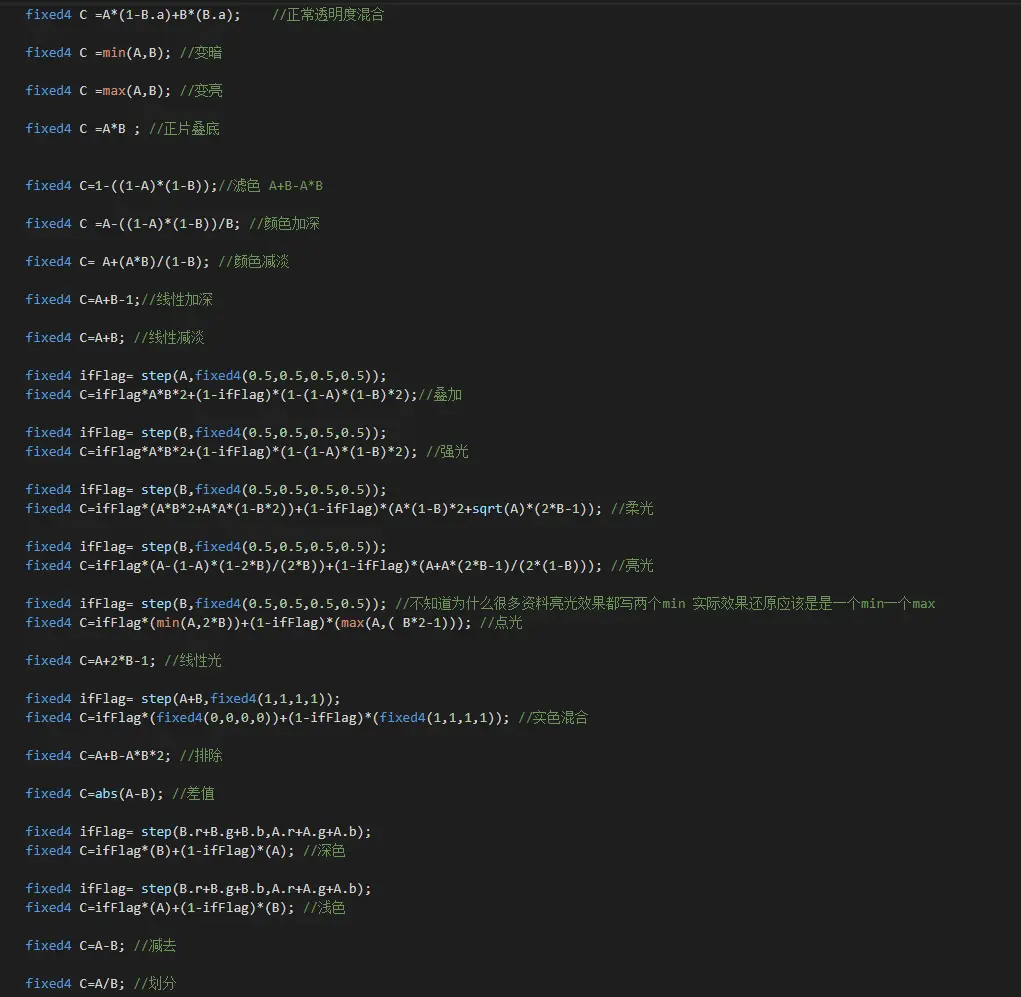# Unity实现与Ps效果对比图

• 创建材质球 给与材质球该shader 将材质球赋给 Image UI 或者 2D Sprite

• 添加对应贴图 如下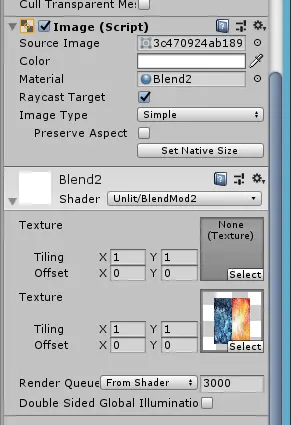• 查看实验效果（左 Unity实现 右 Ps对比）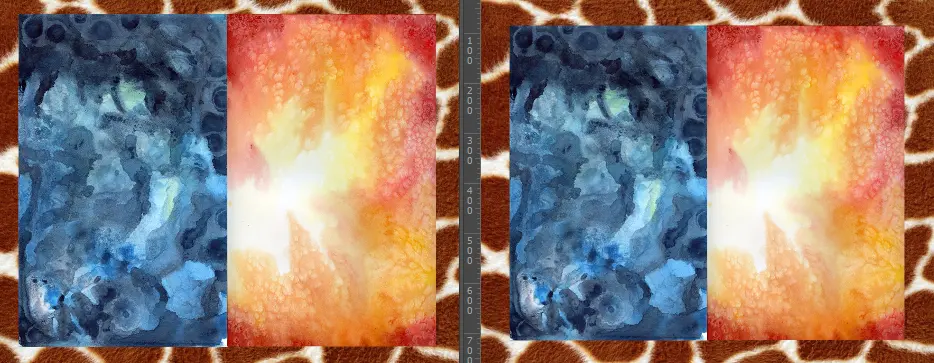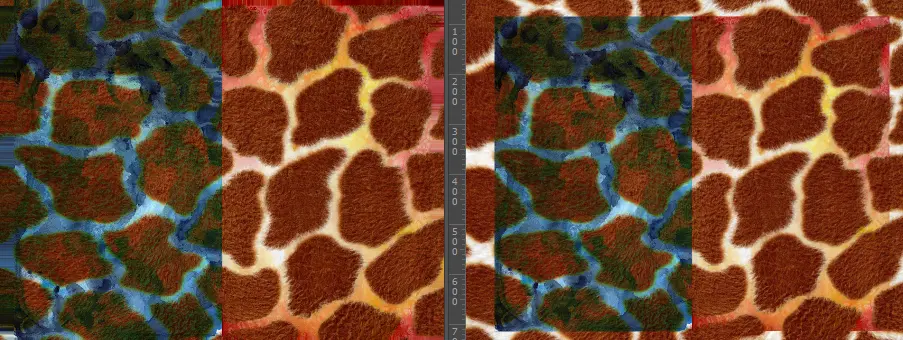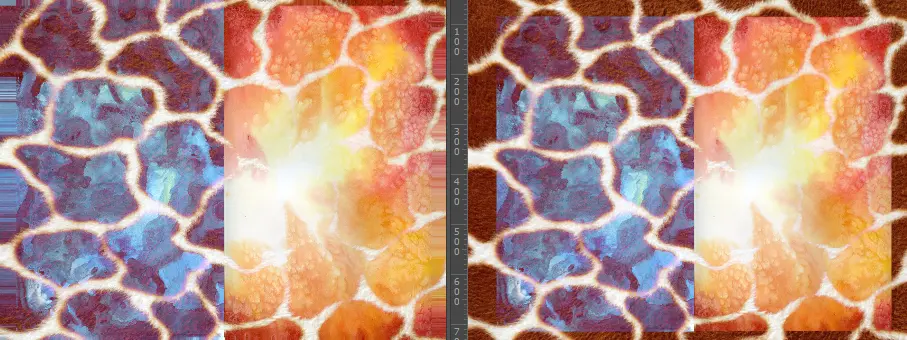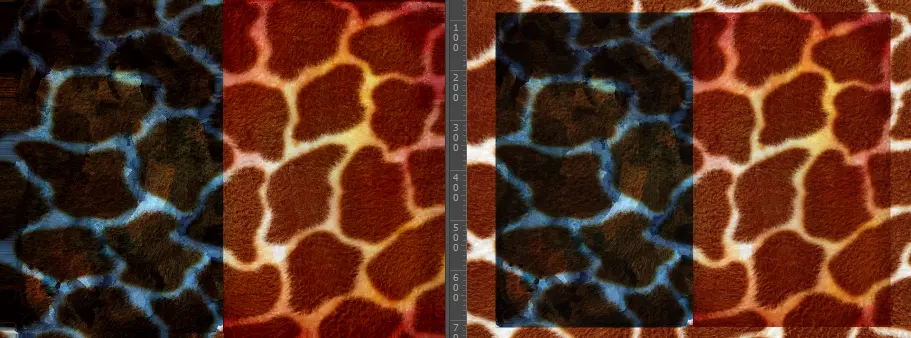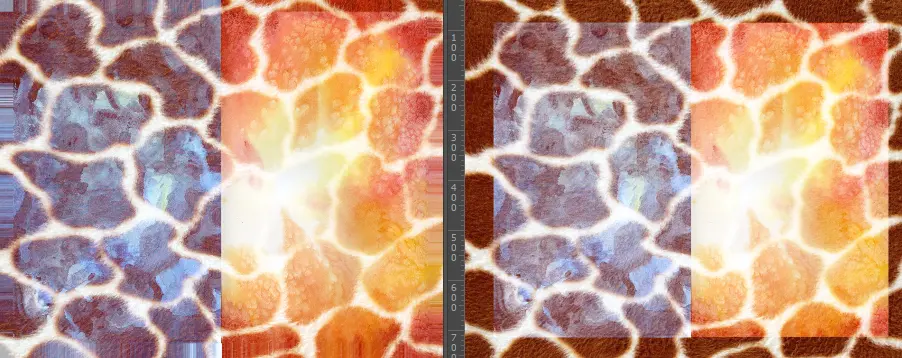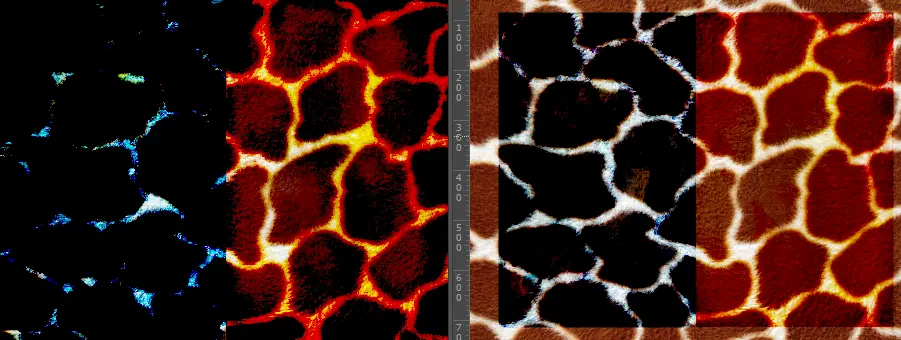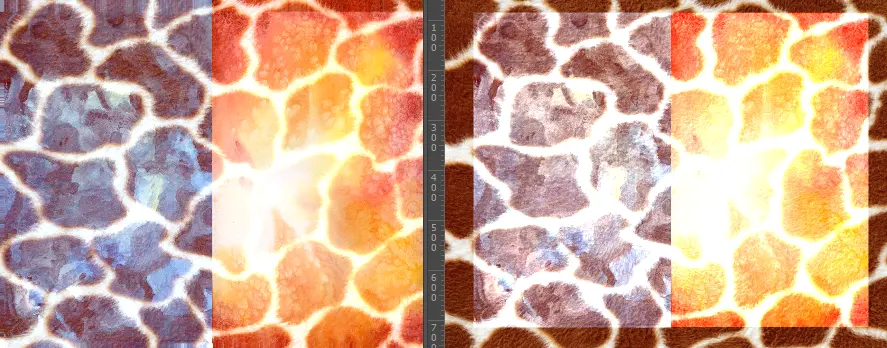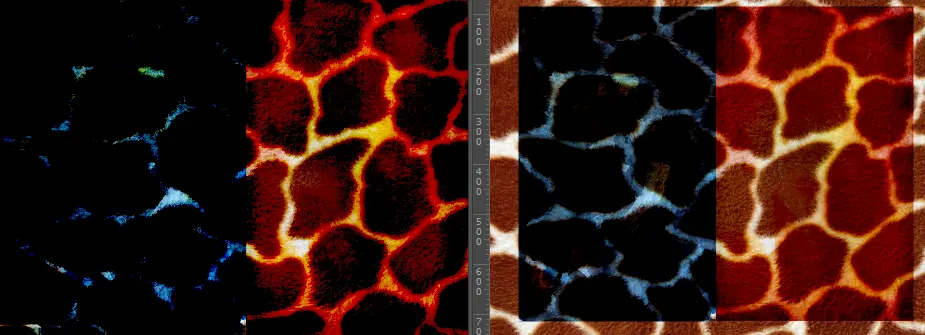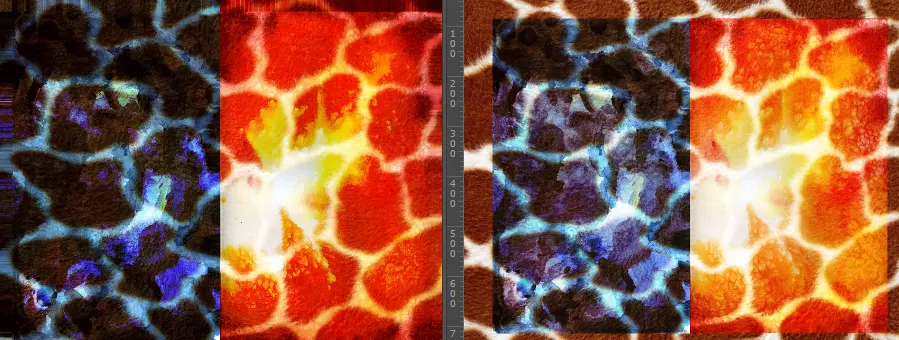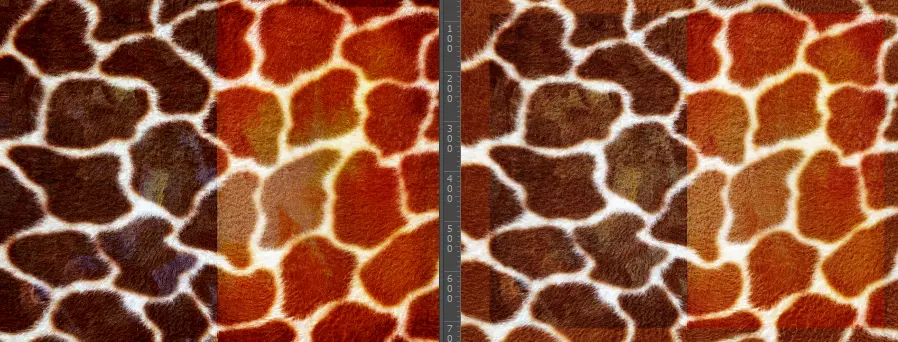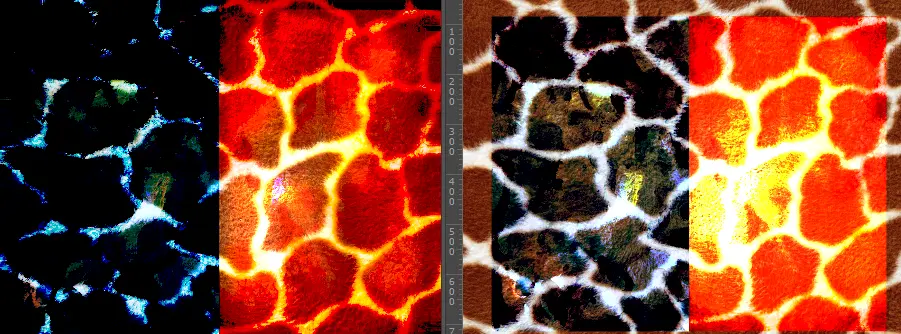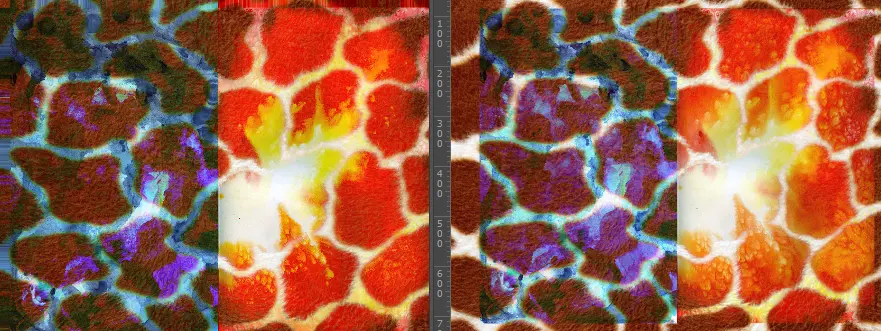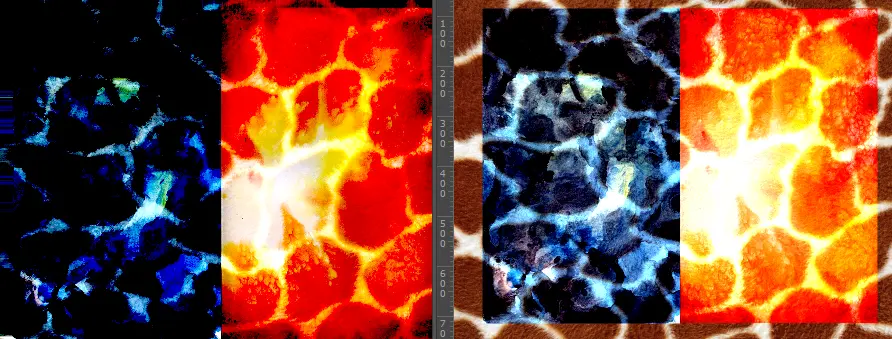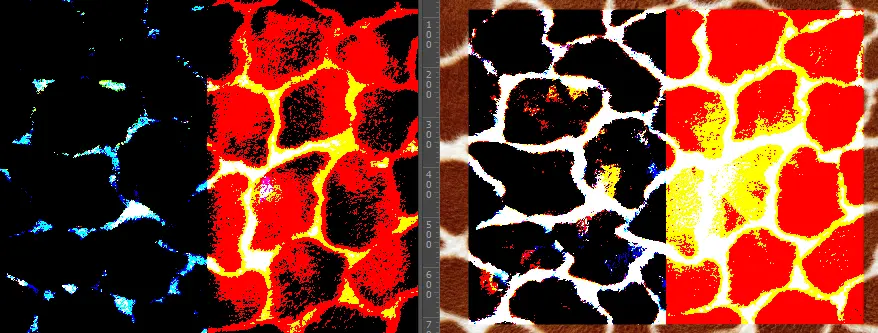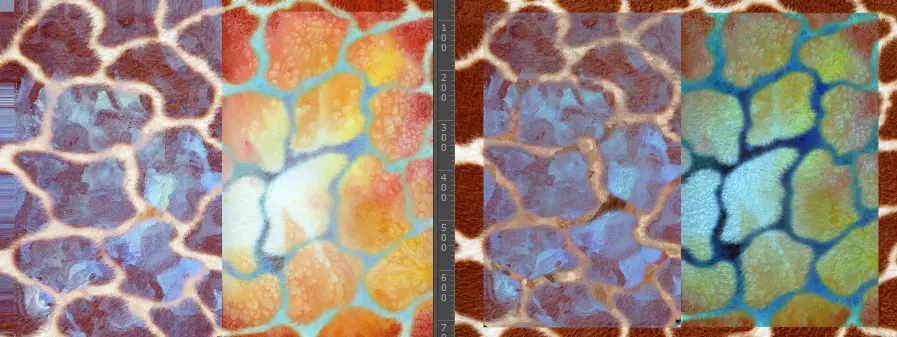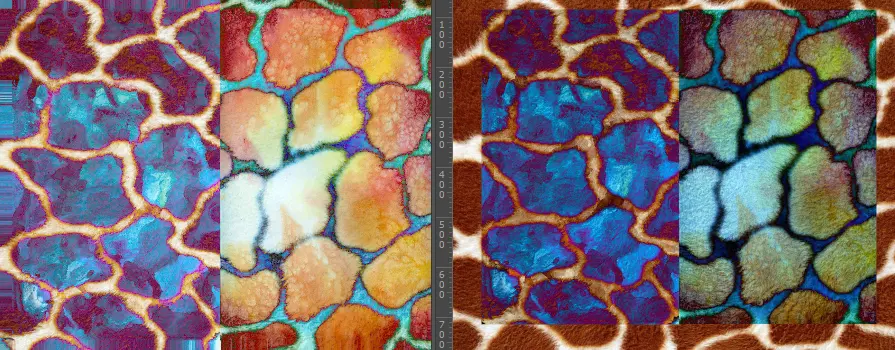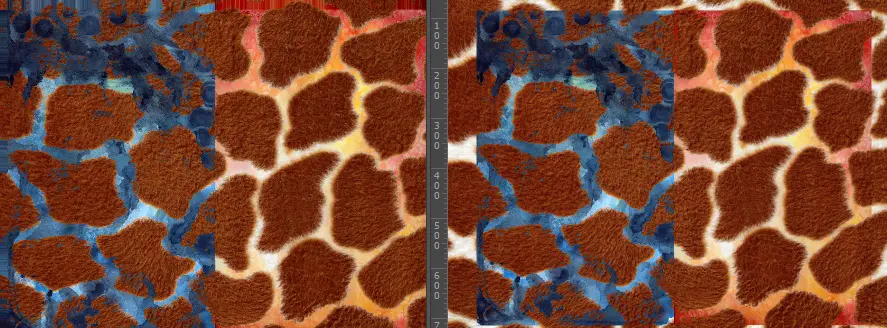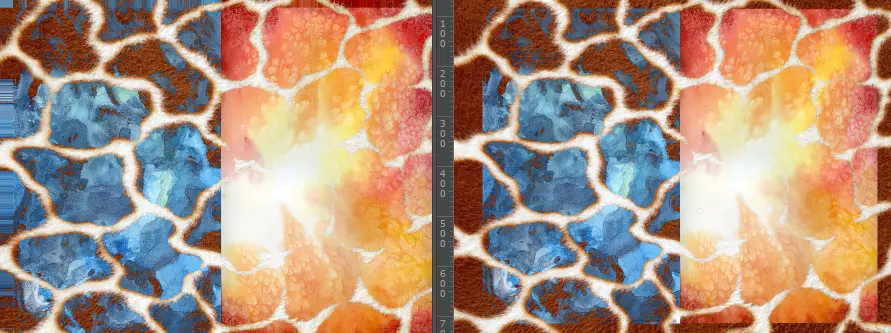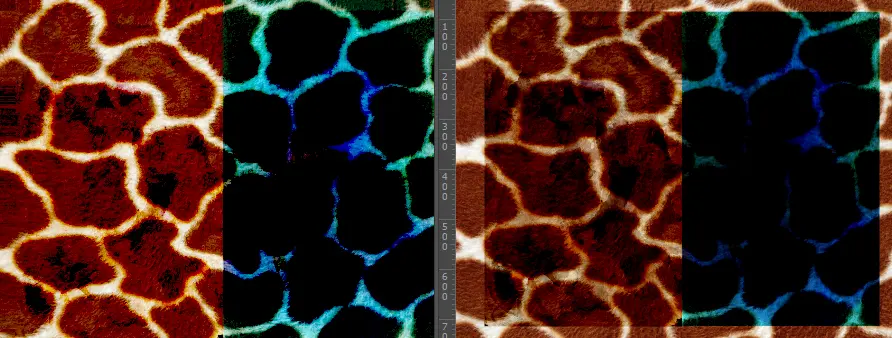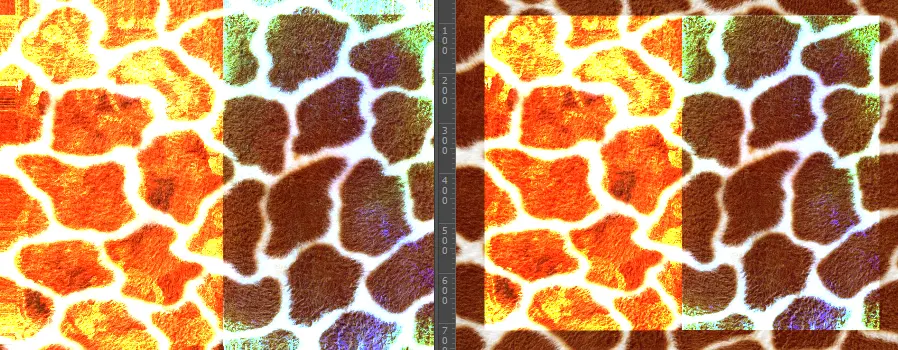# 核心代码

fixed4 C =A*(1-B.a)+B*(B.a);  //正常透明度混合

fixed4 C =min(A,B); //变暗

fixed4 C =max(A,B); //变亮

fixed4 C =A*B ; //正片叠底

fixed4 C=1-((1-A)*(1-B));//滤色 A+B-A*B

fixed4 C =A-((1-A)*(1-B))/B; //颜色加深

fixed4 C= A+(A*B)/(1-B); //颜色减淡

fixed4 C=A+B-1;//线性加深

fixed4 C=A+B; //线性减淡

fixed4 ifFlag= step(A,fixed4(0.5,0.5,0.5,0.5));

fixed4 C=ifFlag*A*B*2+(1-ifFlag)*(1-(1-A)*(1-B)*2);//叠加

fixed4 ifFlag= step(B,fixed4(0.5,0.5,0.5,0.5));

fixed4 C=ifFlag*A*B*2+(1-ifFlag)*(1-(1-A)*(1-B)*2); //强光

fixed4 ifFlag= step(B,fixed4(0.5,0.5,0.5,0.5));

fixed4 C=ifFlag*(A*B*2+A*A*(1-B*2))+(1-ifFlag)*(A*(1-B)*2+sqrt(A)*(2*B-1)); //柔光

fixed4 ifFlag= step(B,fixed4(0.5,0.5,0.5,0.5));

fixed4 C=ifFlag*(A-(1-A)*(1-2*B)/(2*B))+(1-ifFlag)*(A+A*(2*B-1)/(2*(1-B))); //亮光

fixed4 ifFlag= step(B,fixed4(0.5,0.5,0.5,0.5));

fixed4 C=ifFlag*(min(A,2*B))+(1-ifFlag)*(max(A,( B*2-1))); //点光

fixed4 C=A+2*B-1; //线性光

fixed4 ifFlag= step(A+B,fixed4(1,1,1,1));

fixed4 C=ifFlag*(fixed4(0,0,0,0))+(1-ifFlag)*(fixed4(1,1,1,1)); //实色混合

fixed4 C=A+B-A*B*2; //排除

fixed4 C=abs(A-B); //差值

fixed4 ifFlag= step(B.r+B.g+B.b,A.r+A.g+A.b);

fixed4 C=ifFlag*(B)+(1-ifFlag)*(A); //深色

fixed4 ifFlag= step(B.r+B.g+B.b,A.r+A.g+A.b);

fixed4 C=ifFlag*(A)+(1-ifFlag)*(B); //浅色

fixed4 C=A-B; //减去

fixed4 C=A/B; //划分

# 结语

• 这些公式不用记住 再用到的时候查找挑选合适的方法就好